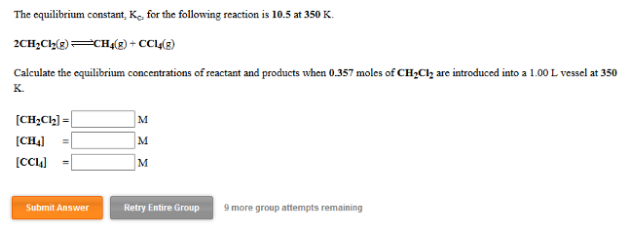# Problem: The equilibrium constant, Kc, for the following reaction is 10.5 at 350 K. 2CH2Cl2 ⇌ CH4 (g) + CCl4 (g) Calculate the equilibrium concentrations of reactant and products when 0.357 moles of CH2Cl2 are introduced into a 1.00 L vessel at 350 K. [CH2Cl2] = __________ M [CH4] =  __________ M[CCl4] = __________  M

###### FREE Expert Solution
96% (293 ratings)###### Problem Details

The equilibrium constant, Kc, for the following reaction is 10.5 at 350 K.

2CH2Cl2 ⇌ CH4 (g) + CCl4 (g)

Calculate the equilibrium concentrations of reactant and products when 0.357 moles of CH2Cl2 are introduced into a 1.00 L vessel at 350 K.

[CH2Cl2] = __________ M

[CH4] =  __________ M

[CCl4] = __________  M